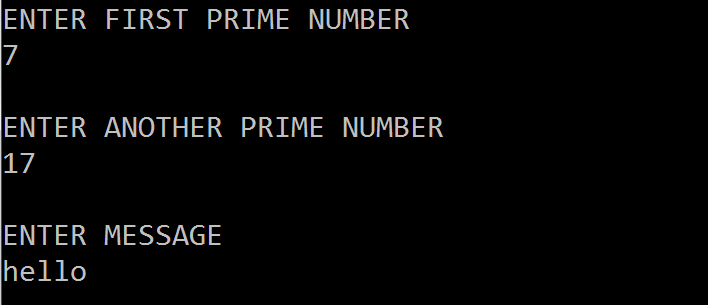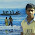# C code to implement RSA Algorithm(Encryption and Decryption)

C program to implement RSA algorithm. The given program will Encrypt and Decrypt a message using RSA Algorithm.

```#include<stdio.h>
#include<conio.h>
#include<stdlib.h>
#include<math.h>
#include<string.h>

long int p,q,n,t,flag,e,d,temp,j,m,en,i;
char msg;
int prime(long int);
void ce();
long int cd(long int);
void encrypt();
void decrypt();
void main()
{
clrscr();
printf("\nENTER FIRST PRIME NUMBER\n");
scanf("%d",&p);
flag=prime(p);
if(flag==0)
{
printf("\nWRONG INPUT\n");
getch();
exit(1);
}
printf("\nENTER ANOTHER PRIME NUMBER\n");
scanf("%d",&q);
flag=prime(q);
if(flag==0||p==q)
{
printf("\nWRONG INPUT\n");
getch();
exit(1);
}
printf("\nENTER MESSAGE\n");
fflush(stdin);
scanf("%s",msg);
for(i=0;msg[i]!=NULL;i++)
m[i]=msg[i];
n=p*q;
t=(p-1)*(q-1);
ce();
printf("\nPOSSIBLE VALUES OF e AND d ARE\n");
for(i=0;i<j-1;i++)
printf("\n%ld\t%ld",e[i],d[i]);
encrypt();
decrypt();
getch();
}
int prime(long int pr)
{
int i;
j=sqrt(pr);
for(i=2;i<=j;i++)
{
if(pr%i==0)
return 0;
}
return 1;
}
void ce()
{
int k;
k=0;
for(i=2;i<t;i++)
{
if(t%i==0)
continue;
flag=prime(i);
if(flag==1&&i!=p&&i!=q)
{
e[k]=i;
flag=cd(e[k]);
if(flag>0)
{
d[k]=flag;
k++;
}
if(k==99)
break;
}
}
}
long int cd(long int x)
{
long int k=1;
while(1)
{
k=k+t;
if(k%x==0)
return(k/x);
}
}
void encrypt()
{
long int pt,ct,key=e,k,len;
i=0;
len=strlen(msg);
while(i!=len)
{
pt=m[i];
pt=pt-96;
k=1;
for(j=0;j<key;j++)
{
k=k*pt;
k=k%n;
}
temp[i]=k;
ct=k+96;
en[i]=ct;
i++;
}
en[i]=-1;
printf("\nTHE ENCRYPTED MESSAGE IS\n");
for(i=0;en[i]!=-1;i++)
printf("%c",en[i]);
}
void decrypt()
{
long int pt,ct,key=d,k;
i=0;
while(en[i]!=-1)
{
ct=temp[i];
k=1;
for(j=0;j<key;j++)
{
k=k*ct;
k=k%n;
}
pt=k+96;
m[i]=pt;
i++;
}
m[i]=-1;
printf("\nTHE DECRYPTED MESSAGE IS\n");
for(i=0;m[i]!=-1;i++)
printf("%c",m[i]);
}
```

Output ff the above program:-RSA InputsRSA Result

Related Programs:-

Encrypt and Decrypt a message using Substitution Cipher

Encrypt and Decrypt a message using Vernan Cipher

Encrypt and Decrypt a message using Transposition Cipher

Encrypt and Decrypt a message using PlayFair Cipher

Calculate compression ratio

1.1.check network label above to access all network programs or check it: http://www.coders-hub.com/2013/04/implementation-of-rsa-using-java.html

2.Can RSA algorithm can be implemented on NS2 by creating the nodes, Can u write from where i get it?

3.great code, thanks so much....

1.4.thank tou so much!!!!

5.thank you so much!!!!!

6.very long code !!!
but it a great code
thanks... :)

7.how can i calculate run time for encryption &decryption......plz help

8.can you pls explain what 'ce' is?
void ce()

1.its a function to compute encryption key e

9.This comment has been removed by the author.

10.can you send me the code for encrypting and decrypting a image using RSA

1.Anonymous2:52 pm

thanks

2.can you send me the code for encrypting and decrypting a image using RSA to kaliraj401@gmail.com

3.can you send me the code for encrypting and decrypting a image using RSA to sean123456p@gmail.com
thanks

11.12.can i have this program in c#

13.code not run on Linux correctly if enter prime 5 ,3 not encrypt correctly

14.hi ,
could you pls let me know how the same code works using fixed block size ? example 4?

15.This comment has been removed by the author.

16.In the encrypt function, why is 96 added to pt, and subsequently added back?

1.I too want to know the same thing.

2.This is based on ascii code. Ascii code of 'A' is 65 and 'a' is 97.

3.Is the code also working without adding/subtracting the 96? Thanks!

4.hi, I want to ask. I have problem when modified your code in c. I have same question, why pt=pt-96 and pt=k+96? i have seen ascii table but the result is false. When i try to calculate, the result between manual and program is different. May you give your email? because i have so many problem and want talk about RSA.

5.This comment has been removed by the author.

6.hi, I want to ask. I have problem when modified your code in c. I have same question, why pt=pt-96 and pt=k+96? i have seen ascii table but the result is false. When i try to calculate, the result between manual and program is different. May you give your email? because i have so many problem and want talk about RSA.

maybe you have modified your code, you can send your code on email oktasafira98@gmail.com, thank you so much and please respond my question

17.Also, is there a limit on how large the prime numbers can be for this method?

18.could you tell me what variable "flag" means?

1.Here we are checking input number is a prime or not and setting 0 or 1 value to flag based on prime number and than doing rest of the things.

19.can you send me the code for encrypting and decrypting a image using RSA to kaliraj401@gmail.com

20.in encrypt() function, what does this part do?
for(j=0;j<key;j++)
{
k=k*pt;
k=k%n;
}

21.Can you please tell me that in the function void ce(), what does the following do:
if(k==99)
break;

22.Anonymous5:50 pm

Hi im having a problem when i run your code, i get this error:

in function 'main':
38 warning: comparison between pointer and integer[enabled by default]
undefined reference to 'clrscr'

23.Anonymous7:22 pm

Hi im having a problem when i run your code, i get this error:

in function 'main':
38 warning: comparison between pointer and integer[enabled by default]
undefined reference to 'clrscr'

24.why pt=pt-96 in this program.plz ..

25.26.i need source code encryption description in gost algorithm, anyone can help me ?

27.can i have a code for secure user authentication system using rsa key generation..?

28.is it possible to make it work using "char temp" instead of "int temp"

29.is there anyway to lock a folder using RSA encryption?

30.if we give prime numbers as 251 & 263 then its not working.. anybody know why...?

31.code is not running it is getting some error in 38th line

1.can you send me the modified code for my mail
jeevanreddy307@gmail.com

32.This comment has been removed by the author.

33.how large primes nos it can handle...
If we want the primes nos to be very large then how to implement it?

34.what is the prime number for?

35.Why do we subtract 96 in encryption and decryption